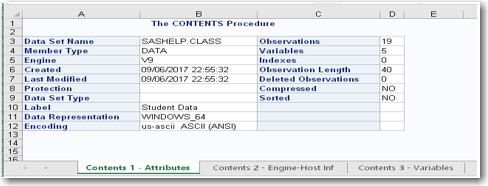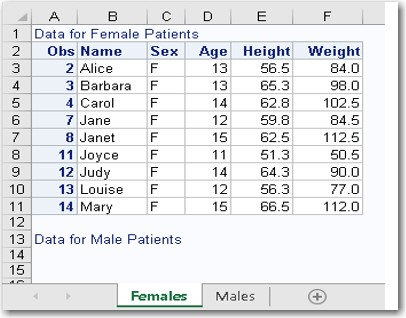View more Problem Solvers posts

The ODS Excel destination, which became a production feature in SAS 9.4M3 (TS1M3), generates Microsoft Excel workbooks in native XLSX format. This destination generates multiple worksheets per workbook with each output object (e.g., a table or graphic) the destination encounters by default. The ODS Excel destination is also flexible, enabling you to vertically control the worksheet and place output objects wherever you want. This blog demonstrates the destination’s flexibility and how you can modify its default behavior by using the ODS EXCEL statement's SHEET_INTERVAL= option.

## Adding tables and graphics on the same Microsoft Excel worksheet

By default, the ODS Excel destination adds a new worksheet for each table and graphic. However, at times, you might not want to use this default behavior. If you want more control over this, the SHEET_INTERVAL= ODS Excel option determines when a new worksheet is created. Valid values for the SHEET_INTERVAL= option include:

• TABLE (the default value) - new sheet for each table in output
• NONE - keep the output that follows on the current sheet
• PAGE - new sheet for each page of SAS output
• PROC - new sheet beginning at the PROC step boundary
• BYGROUP - new sheet for each BY group of output
• NOW - begin a new sheet immediately

The value NOW, new for SAS 9.4M5 (TS1M5), triggers the creation of a new worksheet after the destination encounters the next output object.
As an example of opting not to use the default behavior, consider a case where you have a CONTENTS procedure without any options. This procedure generates three separate worksheets with the data-set attributes, the engine host information, and the variable list.

The following table shows the default output that you receive with three individual worksheets:However, if you want to place all three objects on a single worksheet, you can do that by setting the option SHEET_INTERVAL="NONE". The option setting SHEET_INTERVAL="PROC" could also be used in this example which would create a new worksheet only when a new procedure is encountered.
The following example illustrates how to use this option to include all your output on the same worksheet:

```ods excel file="c:\test.xlsx" options sheet_interval="none"); proc contents data=sashelp.class; run; ods excel close;```

## Adding text and tables to a new worksheet

Two of the most popular ways to add text on worksheets are to use either the ODS TEXT= statement or the ODSTEXT procedure with the Excel destination. The following example adds text to a worksheet by using the ODS TEXT= statement. You include this statement before each PRINT procedure in this example:

```ods excel file="c:\temp\test.xlsx"; ods excel options(sheet_name="Females");   ods text="Data for Female Patients"; proc print data=sashelp.class(where=(sex="F")); run;   ods excel options(sheet_name="Males"); ods text="Data for Male Patients";   proc print data=sashelp.class(where=(sex="M")); run;   ods excel close;```

OutputNotice that the first text string appears at the top of the first worksheet as expected. However, the text from the second ODS TEXT= statement appears at the bottom of this same page rather than at the top of the next worksheet containing the related data table. This behavior illustrates that the ODS TEXT= option is not very flexible. There is no good way to solve this issue.

However, you can use the SAS 9.4 ODSTEXT procedure in combination with the SHEET_INTERVAL= option to move the text string to the appropriate worksheet.
The following example uses PROC ODSTEXT and the SHEET_INTERVAL= option to move the text string "Statistics for Male Patients" to the top of the second worksheet:

```ods excel file="c:\temp\test.xlsx"; ods excel options(sheet_name="Females");   ods text="Data for Female Patients"; proc print data=sashelp.class(where=(sex="F")); run;   ods excel options(sheet_name="Males" sheet_interval="now"); ods excel options(sheet_name="Males" sheet_interval="none");   proc odstext; p "Data for Male Patients"; run;   proc print data=sashelp.class(where=(sex="M")); run;   ods excel close;```

## Adding multiple tables or graphs on the same worksheet

This final example demonstrates how you can use the SHEET_INTERVAL= option to add multiple tables and graphics to the same Excel worksheet. First, we use the SHEET_INTERVAL="NONE" option in the first ODS EXCEL statement to place the first table and graph on the same worksheet. Then, the SHEET_INTERVAL="NOW" option is included in the second ODS EXCEL statement option to create a second worksheet and write the second table and graph to that worksheet:

```ods graphics / height=2.5in width=3.5in; ods excel file="c:\scratch\test.xlsx" options(sheet_interval="none");   proc print data=sashelp.class(where=(sex="F")); run;   proc sgplot data=sashelp.class(where=(sex="F")); scatter x=age y=height; run;   ods excel options(sheet_interval="now");     proc print data=sashelp.class(where=(sex="M")); run;   proc sgplot data=sashelp.class(where=(sex="M")); scatter x=age y=height; run;   ods excel close;```

ShareSAS Technical Support Analyst

Chevell is a Senior Principal Technical Support Analyst in the Foundation SAS group within SAS Technical Support. His main support areas include the Output Delivery System and XML technologies.

### 1 Comment

1.Is it possible to read a formula from Excel into SAS?
Example: Read this from an Excel cell:
=HYPERLINK("https://www.cms.gov/Medicare/Quality-Initiatives-Patient-Assessment-Instruments/Value-Based-Programs/SNF-VBP/Confidential-Feedback-Reporting-and-Review-and-Corrections-", "Confidential Feedback Reporting & Review and Corrections")

This site uses Akismet to reduce spam. Learn how your comment data is processed.